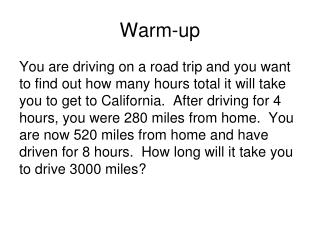DownloadDownload PresentationWarm-up

# Warm-up

Télécharger la présentation## Warm-up

- - - - - - - - - - - - - - - - - - - - - - - - - - - E N D - - - - - - - - - - - - - - - - - - - - - - - - - - -
##### Presentation Transcript

1. Warm-up You are driving on a road trip and you want to find out how many hours total it will take you to get to California. After driving for 4 hours, you were 280 miles from home. You are now 520 miles from home and have driven for 8 hours. How long will it take you to drive 3000 miles?

2. Writing Equations of Lines

3. 3 Forms Slope-Intercept Point-Slope Standard **Always have the variables x & y**

4. Break into groups of 4.

5. Slope Intercept m is the slope of the line b is the y-intercept of the line

6. Example 1) Write the equation of the line that has a slope of 4, and a y-intercept of -2. 2) Write the equation of the line between the points (2, 4) and (4, 12).

7. Example 3) Write the equation of the line pictured.

8. Point-Slope (x1, y1) is a point on the line m is the slope of the line

9. Examples • Write the equation of the line that passes through the point (4, -2) and has a slope of 6. 5) Write the equation of the line that passes through the points (1, 8) and (-2, 6).

10. Standard Form Where A, B & C are integers A is NOT negative

11. Putting Equations into Standard Form 1) Put the equation into slope-intercept form. 2) Subtract mx from both sides (EX: y = 3x + 7 becomes –3x + y = 7) 3) If the number in front of x is negative, multiply everything by -1. 4) If the number in front of x is a fraction, multiply everything by the denominator.

12. Examples • Write the equation of the line that passes through the points (2, 6) and (3, -3) in standard form. • Write the equation of the line that has slope -5 and passes through the point (1, -4) in standard form. • Write the equation of the line through the points (3, -5) and (7, 1) in standard form.

13. Pop Quiz • You are given the slope of a line and a point on the line. How can you find an equation of the line? • You are given the slope of a line and the y-intercept of the line, how can you find an equation of the line? • You are given the x-intercept and the y-intercept of a line, how can you find an equation of the line?

14. WRITE THIS 1) Given slope (m) and y int (b): plug into y = mx + b 2) Given slope (m) and a point (x, y): plug into y = mx + b to find b. Then go to step 1. 3) Given two points (x1,y1) and (x2, y2): First find slope, then go to step 2.

15. Exit Ticket 9) Write the equation of the line perpendicular to the line y = ½ x + 8 through the point (1, -7) in all 3 forms.

16. Flipped

17. Slope Intercept m is the slope of the line b is the y-intercept of the line

18. Example 1) Write the equation of the line that has a slope of 4, and a y-intercept of -2. 2) Write the equation of the line between the points (2, 4) and (4, 12).

19. Example 3) Write the equation of the line pictured.

20. Point-Slope (x1, y1) is a point on the line m is the slope of the line

21. Examples • Write the equation of the line that passes through the point (4, -2) and has a slope of 6. 5) Write the equation of the line that passes through the points (1, 8) and (-2, 6).

22. Standard Form Where A, B & C are integers A is NOT negative

23. Putting Equations into Standard Form 1) Put the equation into slope-intercept form. 2) Subtract mx from both sides (EX: y = 3x + 7 becomes –3x + y = 7) 3) If the number in front of x is negative, multiply everything by -1. 4) If the number in front of x is a fraction, multiply everything by the denominator.

24. Examples • Write the equation of the line that passes through the points (2, 6) and (3, -3) in standard form. • Write the equation of the line that has slope -5 and passes through the point (1, -4) in standard form. • Write the equation of the line through the points (3, -5) and (7, 1) in standard form.

25. Pop Quiz • You are given the slope of a line and a point on the line. How can you find an equation of the line? • You are given the slope of a line and the y-intercept of the line, how can you find an equation of the line? • You are given the x-intercept and the y-intercept of a line, how can you find an equation of the line?